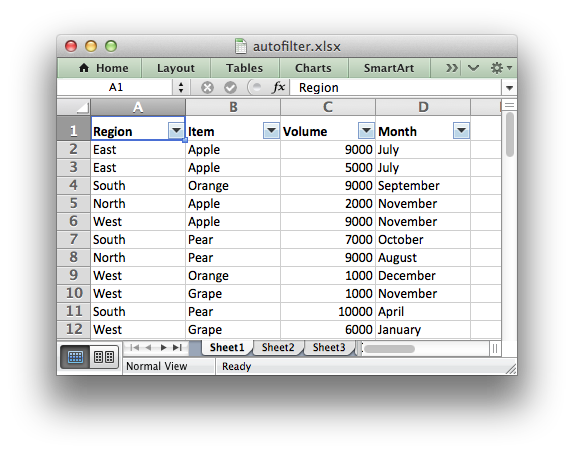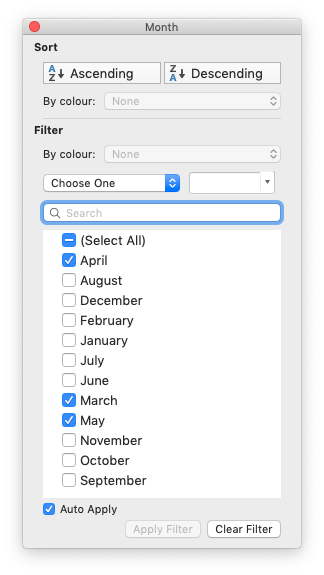libxlsxwriter
Working with Autofilters

An autofilter in Excel is a way of filtering a 2D range of data based on some simple criteria.# Applying an autofilter

The first step is to apply an autofilter to a cell range in a worksheet using the worksheet_autofilter() function:

worksheet_autofilter(worksheet, 0, 0, 10, 3);

Or more explicitly using the RANGE() macro:

worksheet_autofilter(worksheet, RANGE("A1:D11")); //Same as above.

# Filtering data in an autofilter

The `worksheet_autofilter()` function defines the cell range that the filter applies to and creates drop-down selectors in the header row.

However, in order to apply a filter condition it is necessary to add filter rules to the columns using the `worksheet_filter_column()`, `worksheet_filter_column2()` or `worksheet_filter_list()` functions:

• `worksheet_filter_column()`: filter on a single criterion such as "Column == East". More complex conditions such as "<=" or ">=" can also be used.
• `worksheet_filter_column2()`: filter on two criteria such as "Column == East or Column == West". Complex conditions can also be used.
• `worksheet_filter_list()`: filter on a list of values such as "Column in (East, West, North)".

For example you could create a filter like "Column A == East" using a lxw_filter_rule rule and the `worksheet_filter_column()` function like this:

.value_string = "East"};
worksheet_filter_column(worksheet, 0, &filter_rule);

Unfortunately, it isn't sufficient to just specify the filter condition. You must also hide the rows that don't match the criteria since Excel doesn't do that automatically when reading a file. With libxlsxwriter you can hide rows using the `worksheet_set_row_opt()` function with the lxw_row_col_options `hidden` parameter.

The following is an example of how you might filter a data range to match an autofilter criteria:

.value_string = "East"};
worksheet_filter_column(worksheet, 0, &filter_rule);
for (i = 0; i < 100; i++) {
// Write some other cell data for a row...
if (strcmp(data[i].region, "East") == 0) {
// Row matches the filter, no further action required.
}
else {
// Hide rows that don't match the filter.
worksheet_set_row_opt(worksheet, i + 1, LXW_DEF_ROW_HEIGHT, NULL, &hidden);
}
}

Note, the `if()` statement above is written to match the logic of the criteria in the rule. However you could get the same results with the following simpler, but reversed, logic:

if (strcmp(data[i].region, "East") != 0) {
worksheet_set_row_opt(worksheet, i + 1, LXW_DEF_ROW_HEIGHT, NULL, &hidden);
}

# Setting a filter criteria for a column

The `worksheet_filter_column()` and `worksheet_filter_column2()` functions can be used to filter columns in a autofilter range based on simple conditions:

.value_string = "East"};
.value = 3000};
.value = 8000};
worksheet_filter_column (worksheet, 0, &filter_rule1);
worksheet_filter_column2(worksheet, 2, &filter_rule2, &filter_rule3, LXW_FILTER_AND);

The `col` parameter, used in both these functions, is a zero indexed column number and must refer to a column in an existing autofilter created with `worksheet_autofilter()`.

The `criteria` parameter in lxw_filter_rule can have one of the following values:

The `value` parameter is used to set a numeric matching condition while `value_string` is used to set a string matching condition. Excel also allows some simple string matching operations:

// Begins with c.
.value_string = "c*"};
// Doesn't begin with c.
.value_string = "c*"};
// Ends with c.
.value_string = "*c"};
// Doesn't end with c.
.value_string = "*c"};
// Contains c.
.value_string = "*c*"};
// Doesn't contain c.
.value_string = "*c*"};

You can use "*" to match any character or number and "?" to match any single character or number. No other regular expression quantifier is supported by Excel's filters. Excel's regular expression characters can be escaped using "~".

# Setting a column list filter

Prior to Excel 2007 it was only possible to have either 1 or 2 filter conditions such as the ones shown above with the `worksheet_filter_column()` and `worksheet_filter_column()` functions.

Excel 2007 introduced a new list style filter where it is possible to specify one or more "or" style criteria. For example if your column contained data for the months of the year you could filter the data based on certain months:The `worksheet_filter_list()` function can be used to represent these types of filters:

char* list[] = {"March", "April", "May", NULL};
worksheet_filter_list(worksheet, 0, list);

To filter blanks as part of the list use `Blanks` as a list item:

char* list[] = {"March", "April", "May", "Blanks", NULL};
worksheet_filter_list(worksheet, 0, list);

As explained above, it isn't sufficient to just specify filters. You must also hide any rows that don't match the filter condition.

# Example

For a detailed working example with several different filter types see autofilter.c.

worksheet_autofilter
lxw_error worksheet_autofilter(lxw_worksheet *worksheet, lxw_row_t first_row, lxw_col_t first_col, lxw_row_t last_row, lxw_col_t last_col)
Set the autofilter area in the worksheet.
lxw_row_col_options
Options for rows and columns.
Definition: worksheet.h:839
lxw_filter_rule
Options for autofilter rules.
Definition: worksheet.h:1663
LXW_DEF_ROW_HEIGHT
#define LXW_DEF_ROW_HEIGHT
Definition: worksheet.h:76
lxw_filter_rule::criteria
uint8_t criteria
Definition: worksheet.h:1666
worksheet_set_row_opt
lxw_error worksheet_set_row_opt(lxw_worksheet *worksheet, lxw_row_t row, double height, lxw_format *format, lxw_row_col_options *options)
Set the properties for a row of cells.
worksheet_filter_column
lxw_error worksheet_filter_column(lxw_worksheet *worksheet, lxw_col_t col, lxw_filter_rule *rule)
Write a filter rule to an autofilter column.
LXW_FILTER_CRITERIA_GREATER_THAN
@ LXW_FILTER_CRITERIA_GREATER_THAN
Definition: worksheet.h:607
LXW_FILTER_CRITERIA_EQUAL_TO
@ LXW_FILTER_CRITERIA_EQUAL_TO
Definition: worksheet.h:601
RANGE
#define RANGE(range)
Convert an Excel A1:B2 range into a (first_row, first_col, last_row, last_col) sequence.
Definition: utility.h:82
LXW_TRUE
@ LXW_TRUE
Definition: common.h:53
lxw_row_col_options::hidden
uint8_t hidden
Definition: worksheet.h:841
LXW_FILTER_CRITERIA_NOT_EQUAL_TO
@ LXW_FILTER_CRITERIA_NOT_EQUAL_TO
Definition: worksheet.h:604
worksheet_filter_list
lxw_error worksheet_filter_list(lxw_worksheet *worksheet, lxw_col_t col, char **list)
Write multiple string filters to an autofilter column.
worksheet_filter_column2
lxw_error worksheet_filter_column2(lxw_worksheet *worksheet, lxw_col_t col, lxw_filter_rule *rule1, lxw_filter_rule *rule2, uint8_t and_or)
Write two filter rules to an autofilter column.
LXW_FILTER_AND
@ LXW_FILTER_AND
Definition: worksheet.h:635
LXW_FILTER_CRITERIA_LESS_THAN
@ LXW_FILTER_CRITERIA_LESS_THAN
Definition: worksheet.h:610